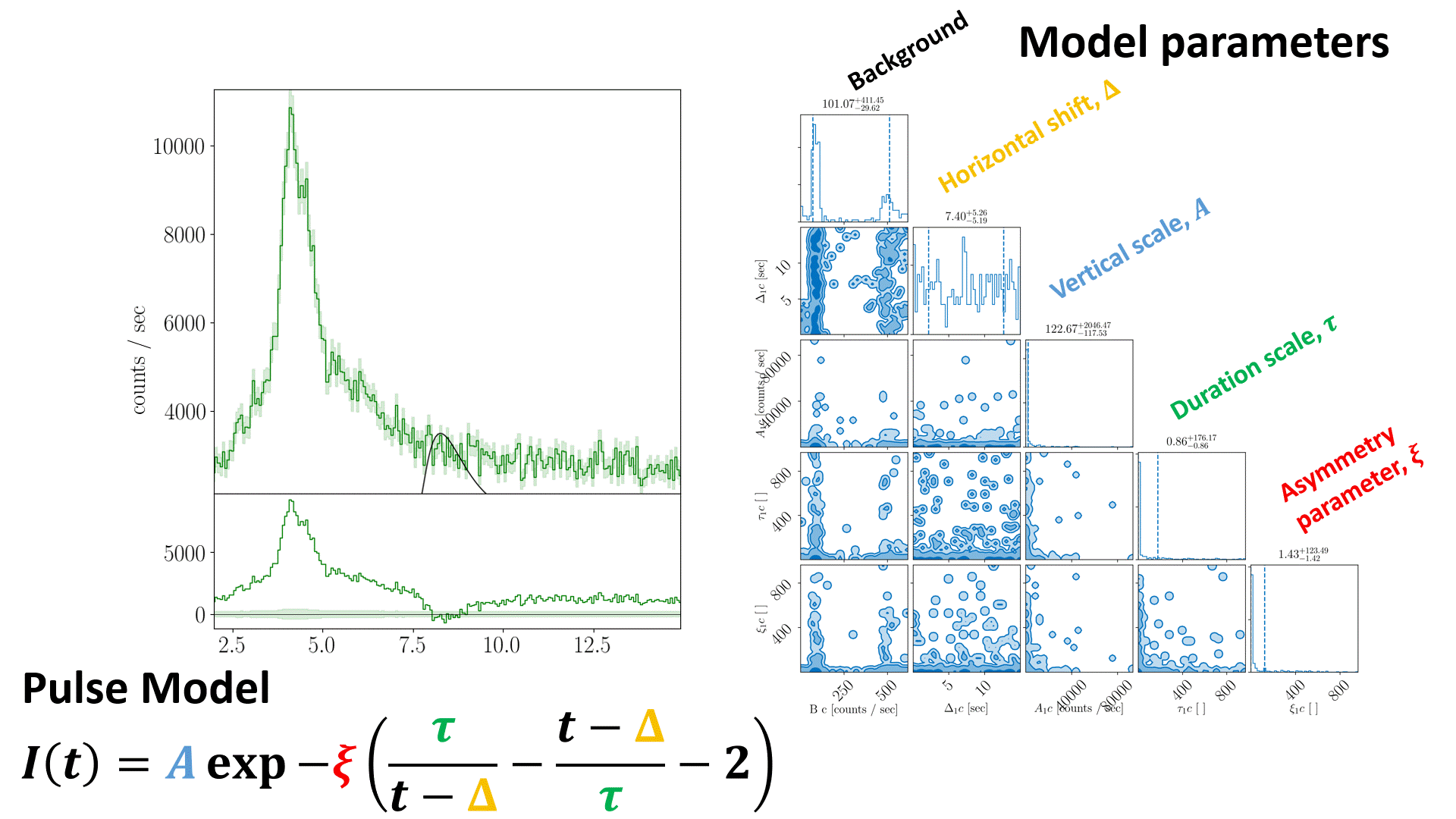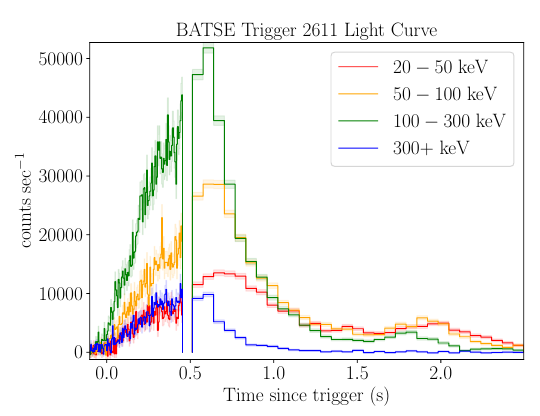# Sampling¶Animation of nested sampling converging on channel 3 of BATSE trigger 8099 with a FRED pulse fit.

The equation in the nested sampling animation should read

$S(t|A,\Delta,\tau,\xi) = A \exp \left[ - \xi \left( \frac{t - \Delta}{\tau} + \frac{\tau}{t-\Delta} \right) -2 \right]$

## Photon Counting¶

Photon emission is a stochastic process. The mean number of photons emitted during time interval $$t$$ is

$\left< N(t) \right> = \lambda t,$

where $$\left< N(t) \right>$$ is the expectation value for $$N$$ over the integration time, with Poisson rate $$\lambda$$. The probability distribution for the random variable $$N$$ is

$\Pr(N(t) = n) = \frac{(\lambda t)^n e^{-\lambda t}}{n!}$

For photons $$\vec{\gamma}=\{\gamma_1, \gamma_2, \ldots, \gamma_n\}$$ emitted via a homogeneous Poisson process ($$\lambda(t)=\lambda$$), then the inter-arrival time between photon $$\gamma_i$$ and $$\gamma_{i+1}$$ is exponentially distributed. The inter-arrival times between photon $$\gamma_i$$ and $$\gamma_{i+k}$$ follow a gamma distribution with

$X \sim \Gamma(k, \lambda^{-1}),$

where $$k=1$$ recovers the exponential distribution

$X \sim \exp (\lambda^{-1}).$

High energy detectors like BATSE accumulate photons at discrete multiples of their clock cycles (sampling frequencies). BATSE has a sampling frequency of 500 kHz (a clock cycle of 2 $${\mu s}$$). These detectors record each photon arrival time (time-tagged event, tte) to the nearest integer multiple of the clock cycle. For BATSE, hardware limitations restricted this to the first 32,768 Large Area Detector (LAD) events, inclusive of all detectors. Only the shortest, moderately bright $${\gamma}$$-ray bursts are contained completely within the tte data. After the tte period has been exhausted, BATSE counts are collected in 64ms intervals (Discriminator Science Data, discsc) for $${\sim 240}$$ seconds after the trigger.BATSE trigger 2611. This is a short bright $${\gamma}$$-ray burst for which the tte data cuts out before the event is over.

### Dead Time¶

BATSE has a small dead time after each photon count of approximately one clock cycle. This dead time is proportional to the energy of the incident photon (or particle event) which triggered the count .

$\tau \sim \alpha \ln \frac{E_{\gamma}}{E_0}$

Where $$E_{\gamma}$$ is the energy of the incident photon, $$E_0= 5.5$$ keV is the reset level of the detector, and $${\alpha}=0.75$$ $${\mu s}$$ is the signal decay time . This means that photon counting is not a true Poisson process when the count rate approaches the sampling frequency. Rather, it follows a truncated Poisson distribution.